﻿Ultimate Oscillator

# Ultimate Oscillator

UltimateOscillator uses a weighted total of three oscillators with different calculation periods. The oscillator uses three time spaces that can be set manually. Default values for these periods are 7, 14 and 28 periods. Note that longer periods are comprised of shorter ones.  That means that 28-period values discount 14-period and 7-period values. Therefore, we use the values of the shortest period three times, so these values influence the result of the oscillator the most.Market Signals

Typically UltimateOscillator generates a buy signal when it falls below 30 and a sell signal when it rises above 70.Calculation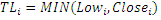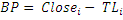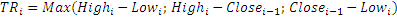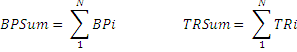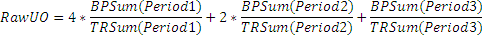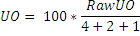Chart Example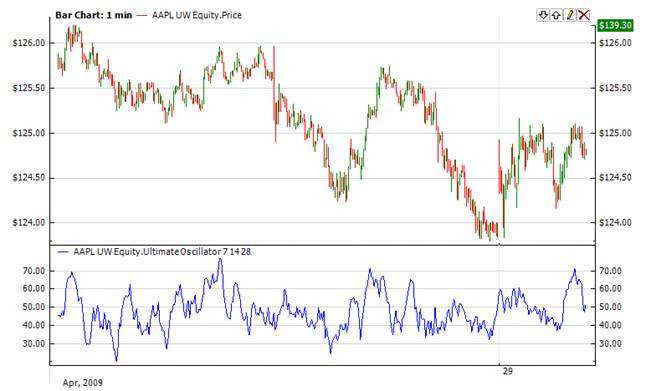Implementation and Usage

To initialize UltimateOscillator use one of the following constructors:

UltimateOscillator – sets default values: period1 = 7, period2 = 14, period3 = 28

UltimateOscillator(Int32, Int32, Int32) – sets values for periods

UltimateOscillator(TimeSpan, TimeSpan, TimeSpan) – sets time periods

Use

OscillatorValue - property to get current valueExample
C#
``` 1// Create new instance
2UltimateOscillator ultOscillator = new UltimateOscillator(14, 14, 28);
3
4// Number of stored values
5ultOscillator.HistoryCapacity = 2;
6